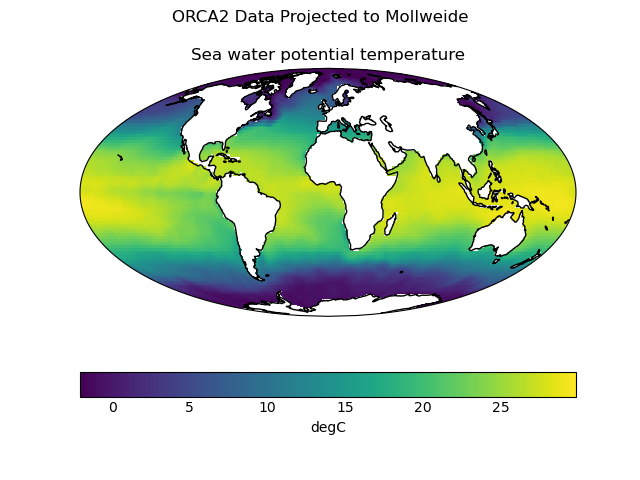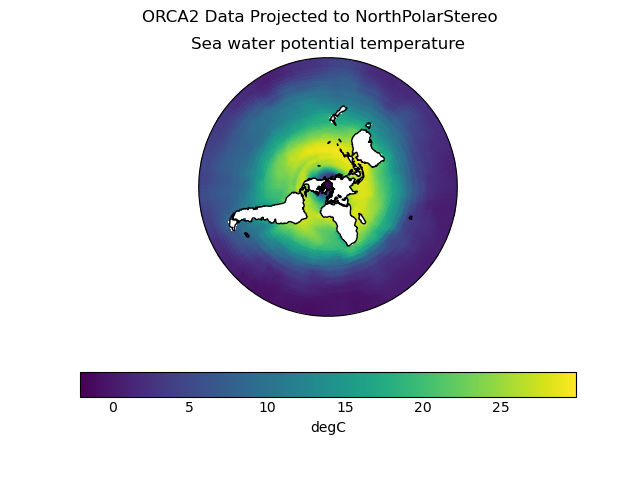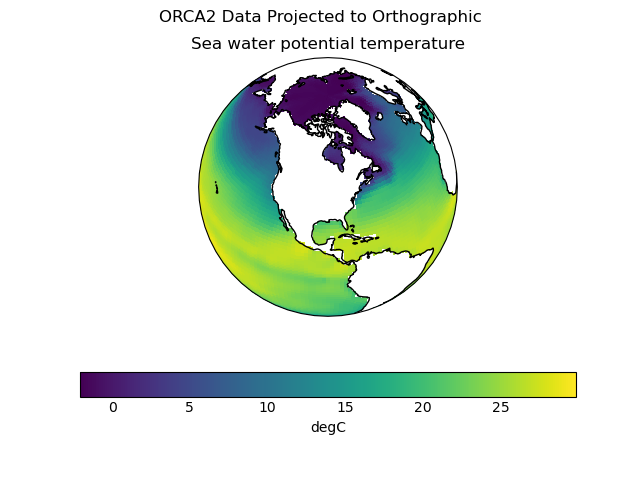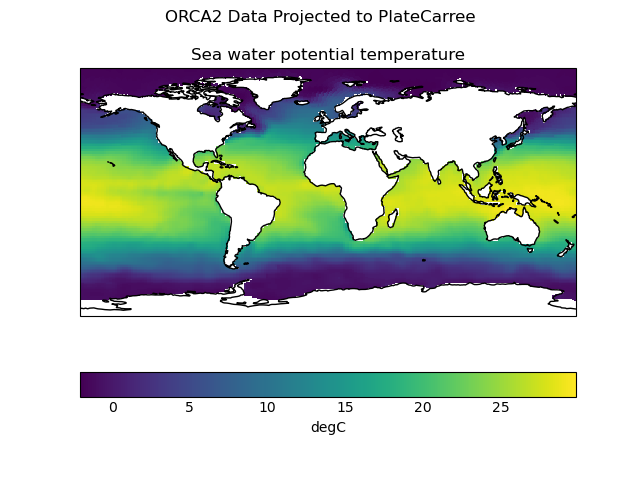# Tri-Polar Grid Projected Plotting#

This example demonstrates cell plots of data on the semi-structured ORCA2 model grid.

First, the data is projected into the PlateCarree coordinate reference system.

Second four pcolormesh plots are created from this projected dataset, using different projections for the output image.

••••```import cartopy.crs as ccrs
import matplotlib.pyplot as plt

import iris
import iris.analysis.cartography
import iris.plot as iplt
import iris.quickplot as qplt

def main():
filepath = iris.sample_data_path("orca2_votemper.nc")

# Choose plot projections
projections = {}
projections["Mollweide"] = ccrs.Mollweide()
projections["PlateCarree"] = ccrs.PlateCarree()
projections["NorthPolarStereo"] = ccrs.NorthPolarStereo()
projections["Orthographic"] = ccrs.Orthographic(
central_longitude=-90, central_latitude=45
)

pcarree = projections["PlateCarree"]
# Transform cube to target projection
new_cube, extent = iris.analysis.cartography.project(
cube, pcarree, nx=400, ny=200
)

# Plot data in each projection
for name in sorted(projections):
fig = plt.figure()
fig.suptitle("ORCA2 Data Projected to {}".format(name))
# Set up axes and title
ax = plt.subplot(projection=projections[name])
# Set limits
ax.set_global()
# plot with Iris quickplot pcolormesh
qplt.pcolormesh(new_cube)
# Draw coastlines
ax.coastlines()

iplt.show()

if __name__ == "__main__":
main()
```

Total running time of the script: ( 0 minutes 3.526 seconds)

Gallery generated by Sphinx-Gallery